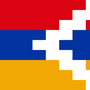1. Forum
2. >
3. Topic: Turkish
4. >
5. "Üç kere beş"

# "Üçkerebeş"

## Translation:Three times five

March 24, 2015Does kere mean times as in "multiplied by" in addition to times as in "it happened 4 times"?Both. It's used in the exact same way you would use "time/times" in English.Yep. "Dört kere oldu" = It's happened four times. "Kez" is also used in that way and is probably slightly preferable.You can only use 'kere' in multiplication but other than they are almost interchangable.Kere, defa, kez, and sefer can all be used to refer to a number of times something occurs (frequency). But of those 4 options, only kere can be used for multiplication.

Do I understand correctly?I didn't mean in multiplication. What's the difference in generalPlus
•997

Could this have a non-mathematical meaning (for example "555" in a phone number)?

Does "kere" always go between the two numbers?

What is the equivalent for more than two numbers (for example how would you translate "the product of two, three and five" into Turkish)?You wouldn't use this in a phone number.

It doesn't have to go between two numbers(Topa üç kere vurdum-I hit the ball three times).

And it would be translated as ''iki, üç ve beşin çarpımı''.•217

Should 3 x 5 be accepted?

We specifically use çarpı for mathematical purposes but kere can also be used informally in math.

Üç çarpı beş (mathematical) -> Üç kere beş (daily)